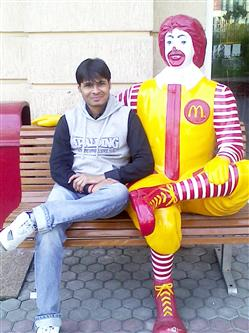EG PAGE
 Login Q&A Books Interest

### Which one is the wrong in the series? 93, 309, 434, 498, 521, 533.

 A: 309 B: 521 C: 498 D: None of these. Your Answer: ---Select--- A B C D To Submit your answer you need to Login Or Register.

### Description:

93-309=216 = 6^3
309-434=125 =5^3
434-498=64 = 4^3
498-521=23 = 3^3
521-533=12 = 2^3

this should be
93-309=216 = 6^3
309-434=125 =5^3
434-498=64 = 4^3
498-525=27 = 3^3
525-533=8 = 2^3,

So the answer is B:521
To comment here,
you need to Login or RegisterPUSHPENDRA YADAV answers with (B)BHAVESH VASOYA answers with (C)SHAILENDRA YADAV answers with (B)
Other Questions by reasoning
 ---> Mathematics : Logic : Science : ??
 ---> January : November :: Sunday : ??
 ---> Dog : Bark :: Goat : ??
 ---> Butter : Milk :: Book : ??
 ---> Genuine : Authentic :: Mirage : ??
 See All